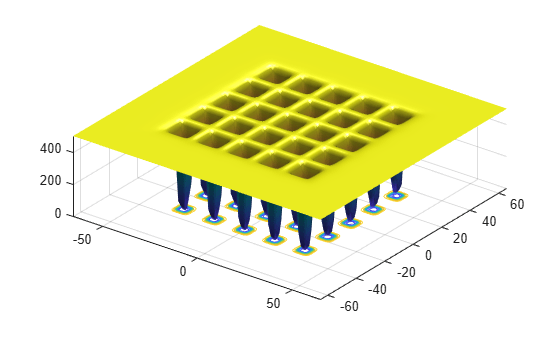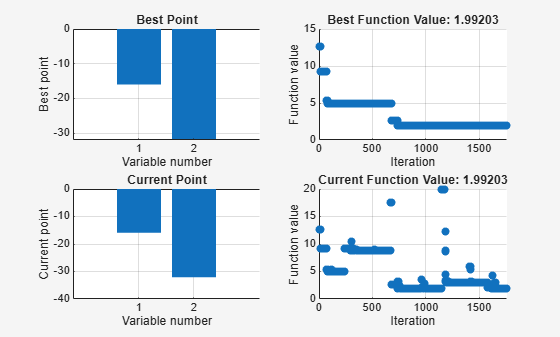Documentation

# simulannealbnd

Find minimum of function using simulated annealing algorithm

## Syntax

``x = simulannealbnd(fun,x0)``
``x = simulannealbnd(fun,x0,lb,ub)``
``x = simulannealbnd(fun,x0,lb,ub,options)``
``x = simulannealbnd(problem)``
``````[x,fval] = simulannealbnd(___)``````
``````[x,fval,exitflag,output] = simulannealbnd(___)``````

## Description

example

````x = simulannealbnd(fun,x0)` finds a local minimum, `x`, to the function handle `fun` that computes the values of the objective function. `x0` is an initial point for the simulated annealing algorithm, a real vector. NotePassing Extra Parameters (Optimization Toolbox) explains how to pass extra parameters to the objective function, if necessary. ```

example

````x = simulannealbnd(fun,x0,lb,ub)` defines a set of lower and upper bounds on the design variables in `x`, so that the solution is always in the range `lb `≤` x `≤` ub`. If `x(i)` is unbounded below, set ```lb(i) = -Inf```, and if `x(i)` is unbounded above, set `ub(i) = Inf`.```

example

````x = simulannealbnd(fun,x0,lb,ub,options)` minimizes with the optimization options specified in `options`. Create `options` using `optimoptions`. If no bounds exist, set `lb = []` and/or `ub = []`.```
````x = simulannealbnd(problem)` finds the minimum for `problem`, where `problem` is a structure described in . Create the `problem` structure by exporting a problem from Optimization app, as described in Exporting Your Work (Optimization Toolbox).```
``````[x,fval] = simulannealbnd(___)```, for any syntax, returns the value of the objective function `fun` at the solution `x`.```

example

``````[x,fval,exitflag,output] = simulannealbnd(___)``` additionally returns a value `exitflag` that describes the exit condition of `simulannealbnd`, and a structure `output` with information about the optimization process.```

## Examples

collapse all

Minimize De Jong's fifth function, a two-dimensional function with many local minima.

Plot De Jong's fifth function.

`dejong5fcn`Minimize De Jong's fifth function using `simulannealbnd` starting from the point `[0,0]`.

```fun = @dejong5fcn; x0 = [0 0]; x = simulannealbnd(fun,x0)```
```Optimization terminated: change in best function value less than options.FunctionTolerance. ```
```x = 1×2 -32.0285 -0.1280 ```

The `simulannealbnd` algorithm uses the MATLAB® random number stream, so you might obtain a different result.

Minimize De Jong’s fifth function within a bounded region.

Plot De Jong's fifth function.

`dejong5fcn`Start `simulannealbnd` starting at the point `[0,0]`, and set lower bounds of -64 and upper bounds of 64 on each component.

```fun = @dejong5fcn; x0 = [0 0]; lb = [-64 -64]; ub = [64 64]; x = simulannealbnd(fun,x0,lb,ub)```
```Optimization terminated: change in best function value less than options.FunctionTolerance. ```
```x = 1×2 -15.9790 -31.9593 ```

The `simulannealbnd` algorithm uses the MATLAB® random number stream, so you might obtain a different result.

Observe the progress of `simulannealbnd` by setting options to use some plot functions.

Set simulated annealing options to use several plot functions.

```options = optimoptions('simulannealbnd','PlotFcns',... {@saplotbestx,@saplotbestf,@saplotx,@saplotf});```

Start `simulannealbnd` starting at the point `[0,0]`, and set lower bounds of -64 and upper bounds of 64 on each component.

```rng default % For reproducibility fun = @dejong5fcn; x0 = [0,0]; lb = [-64,-64]; ub = [64,64]; x = simulannealbnd(fun,x0,lb,ub,options)``````Optimization terminated: change in best function value less than options.FunctionTolerance. ```
```x = 1×2 -15.9790 -31.9593 ```

Obtain all the outputs of a simulated annealing minimization.

Plot De Jong's fifth function.

`dejong5fcn`Start `simulannealbnd` starting at the point `[0,0]`, and set lower bounds of -64 and upper bounds of 64 on each component.

```fun = @dejong5fcn; x0 = [0,0]; lb = [-64,-64]; ub = [64,64]; [x,fval,exitflag,output] = simulannealbnd(fun,x0,lb,ub)```
```Optimization terminated: change in best function value less than options.FunctionTolerance. ```
```x = 1×2 -15.9790 -31.9593 ```
```fval = 1.9920 ```
```exitflag = 1 ```
```output = struct with fields: iterations: 1762 funccount: 1779 message: 'Optimization terminated: change in best function value less than options.FunctionTolerance.' rngstate: [1x1 struct] problemtype: 'boundconstraints' temperature: [2x1 double] totaltime: 1.3331 ```

The `simulannealbnd` algorithm uses the MATLAB® random number stream, so you might obtain a different result.

## Input Arguments

collapse all

Function to be minimized, specified as a function handle or function name.`fun` is a function that accepts a vector `x` and returns a real scalar `f`, the objective function evaluated at `x`.

`fun` can be specified as a function handle for a file:

`x = simulannealbnd(@myfun,x0)`

where `myfun` is a MATLAB® function such as

```function f = myfun(x) f = ... % Compute function value at x```

`fun` can also be a function handle for an anonymous function:

`x = simulannealbnd(@(x)norm(x)^2,x0,lb,ub);`

Example: `fun = @(x)sin(x(1))*cos(x(2))`

Data Types: `char` | `function_handle` | `string`

Initial point, specified as a real vector. `simulannealbnd` uses the number of elements in `x0` to determine the number of variables that `fun` accepts.

Example: `x0 = [1,2,3,4]`

Data Types: `double`

Lower bounds, specified as a real vector or real array. If the number of elements in `x0` is equal to that of `lb`, then `lb` specifies that

`x(i) >= lb(i)` for all `i`.

If `numel(lb) < numel(x0)`, then `lb` specifies that

`x(i) >= lb(i)` for ```1 <= i <= numel(lb)```.

In this case, solvers issue a warning.

Example: To specify that all control variables are positive, ```lb = zeros(size(x0))```

Data Types: `double`

Upper bounds, specified as a real vector or real array. If the number of elements in `x0` is equal to that of `ub`, then `ub` specifies that

`x(i) <= ub(i)` for all `i`.

If `numel(ub) < numel(x0)`, then `ub` specifies that

`x(i) <= ub(i)` for ```1 <= i <= numel(ub)```.

In this case, solvers issue a warning.

Example: To specify that all control variables are less than one, `ub = ones(size(x0))`

Data Types: `double`

Optimization options, specified as an object returned by `optimoptions` or a structure. For details, see Simulated Annealing Options.

`optimoptions` hides the options listed in italics; see Options that optimoptions Hides.

`{}` denotes the default value. See option details in Simulated Annealing Options.

OptionDescriptionValues

`AcceptanceFcn`

Function the algorithm uses to determine if a new point is accepted. Specify as `'acceptancesa'` or a function handle.

Function handle | `{'acceptancesa'}`

`AnnealingFcn`

Function the algorithm uses to generate new points. Specify as a name of a built-in annealing function or a function handle.

Function handle | function name | `'annealingboltz'` | `{'annealingfast'}`

`DataType`

Type of decision variable

`'custom'` | `{'double'}`

`Display`

Level of display

`'off'` | `'iter'` | `'diagnose'` | `{'final'}`

DisplayInterval

Interval for iterative display

Positive integer | `{10}`

`FunctionTolerance`

Termination tolerance on function value

For an options structure, use `TolFun`.

Positive scalar | `{1e-6}`

`HybridFcn`

Automatically run `HybridFcn` (another optimization function) during or at the end of iterations of the solver. Specify as a name or a function handle.

```'fminsearch' | 'patternsearch' | 'fminunc' | 'fmincon' | {[]}```

or

1-by-2 cell array | `{@solver, hybridoptions}`, where ```solver = fminsearch```, `patternsearch`, `fminunc`, or `fmincon` `{[]}`

HybridInterval

Interval (if not `'end'` or `'never'`) at which `HybridFcn` is called

Positive integer | `'never'` | `{'end'}`

`InitialTemperature`

Initial value of temperature

Positive scalar | positive vector | `{100}`

`MaxFunctionEvaluations`

Maximum number of objective function evaluations allowed

For an options structure, use `MaxFunEvals`.

Positive integer | `{3000*numberOfVariables}`

`MaxIterations`

Maximum number of iterations allowed

For an options structure, use `MaxIter`.

Positive integer | `{Inf}`

`MaxStallIterations`

Number of iterations over which average change in fitness function value at current point is less than `options.FunctionTolerance`

For an options structure, use `StallIterLimit`.

Positive integer | `{500*numberOfVariables}`

`MaxTime`

The algorithm stops after running for `MaxTime` seconds

For an options structure, use `TimeLimit`.

Positive scalar | `{Inf}`

`ObjectiveLimit`

Minimum objective function value desired

Scalar | `{-Inf}`

`OutputFcn`

Function(s) get(s) iterative data and can change options at run time

For an options structure, use `OutputFcns`.

Function handle | cell array of function handles | `{[]}`

`PlotFcn`

Plot function(s) called during iterations

For an options structure, use `PlotFcns`.

Function handle | built-in plot function name | cell array of function handles | cell array of built-in plot function names | `'saplotbestf'` | `'saplotbestx'` | `'saplotf'` | `'saplotstopping'` | `'saplottemperature'` | `{[]}`

PlotInterval

Plot functions are called at every interval

Positive integer | `{1}`

`ReannealInterval`

Reannealing interval

Positive integer | `{100}`

`TemperatureFcn`

Function used to update temperature schedule

Function handle | built-in temperature function name | `'temperatureboltz'` | `'temperaturefast'` | `{'temperatureexp'}`

Example: `options = optimoptions(@simulannealbnd,'MaxIterations',150)`

Data Types: `struct`

Problem structure, specified as a structure with the following fields:

• `objective` — Objective function

• `x0` — Starting point

• `lb` — Lower bound for `x`

• `ub` — Upper bound for `x`

• `solver``'simulannealbnd'`

• `options` — Options created with `optimoptions` or an options structure

• `rngstate` — Optional field to reset the state of the random number generator

Create the structure `problem` by exporting a problem from the Optimization app, as described in Importing and Exporting Your Work (Optimization Toolbox).

### Note

`problem` must have all the fields as specified above.

Data Types: `struct`

## Output Arguments

collapse all

Solution, returned as a real vector. The size of `x` is the same as the size of `x0`. Typically, `x` is a local solution to the problem when `exitflag` is positive.

Objective function value at the solution, returned as a real number. Generally, `fval` = `fun(x)`.

Reason `simulannealbnd` stopped, returned as an integer.

Exit FlagMeaning
`1`

Average change in the value of the objective function over `options.MaxStallIterations` iterations is less than `options.FunctionTolerance`.

`5`

`options.ObjectiveLimit` limit reached.

`0`

Maximum number of function evaluations or iterations reached.

`-1`

Optimization terminated by an output function or plot function.

`-2`

No feasible point found.

`-5`

Time limit exceeded.

Information about the optimization process, returned as a structure with fields:

• `problemtype` — Type of problem: unconstrained or bound constrained.

• `iterations` — The number of iterations computed.

• `funccount` — The number of evaluations of the objective function.

• `message` — The reason the algorithm terminated.

• `temperature` — Temperature when the solver terminated.

• `totaltime` — Total time for the solver to run.

• `rngstate` — State of the MATLAB random number generator, just before the algorithm started. You can use the values in `rngstate` to reproduce the output of `simulannealbnd`. See Reproduce Your Results.

Watch now